# Chapter 22 Electric Potential Electrical Potential Energy The

• Slides: 34Chapter 22 Electric Potential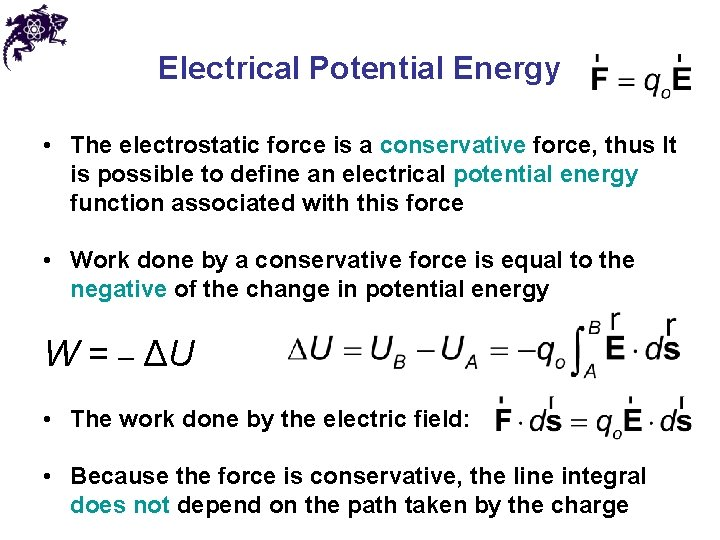Electrical Potential Energy • The electrostatic force is a conservative force, thus It is possible to define an electrical potential energy function associated with this force • Work done by a conservative force is equal to the negative of the change in potential energy W = – ΔU • The work done by the electric field: • Because the force is conservative, the line integral does not depend on the path taken by the chargeElectric Potential • The potential energy per unit charge, U/qo, is the electric potential • Both electrical potential energy and potential are scalar quantities • The potential is characteristic of the field only (independent of the value of qo) and has a value at every point in an electric field • As a charged particle moves in an electric field, it will experience a change in potential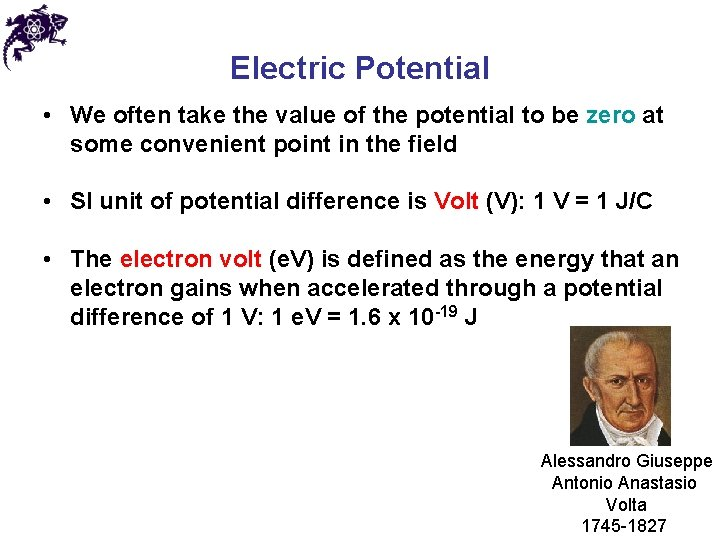Electric Potential • We often take the value of the potential to be zero at some convenient point in the field • SI unit of potential difference is Volt (V): 1 V = 1 J/C • The electron volt (e. V) is defined as the energy that an electron gains when accelerated through a potential difference of 1 V: 1 e. V = 1. 6 x 10 -19 J Alessandro Giuseppe Antonio Anastasio Volta 1745 -1827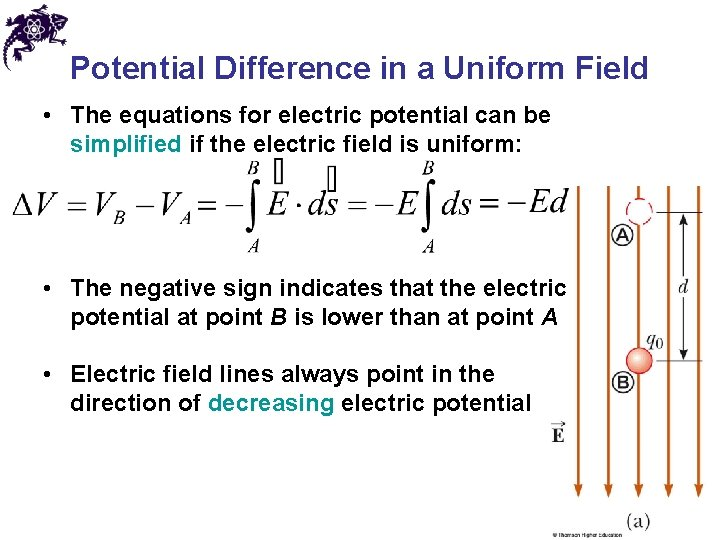Potential Difference in a Uniform Field • The equations for electric potential can be simplified if the electric field is uniform: • The negative sign indicates that the electric potential at point B is lower than at point A • Electric field lines always point in the direction of decreasing electric potential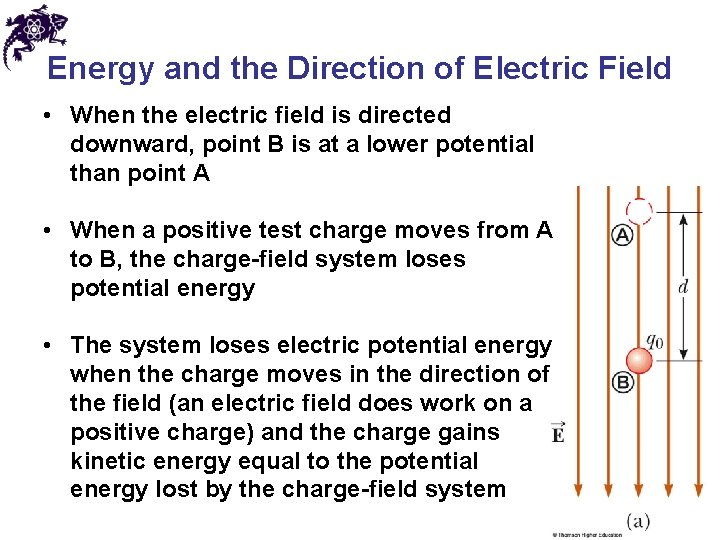Energy and the Direction of Electric Field • When the electric field is directed downward, point B is at a lower potential than point A • When a positive test charge moves from A to B, the charge-field system loses potential energy • The system loses electric potential energy when the charge moves in the direction of the field (an electric field does work on a positive charge) and the charge gains kinetic energy equal to the potential energy lost by the charge-field system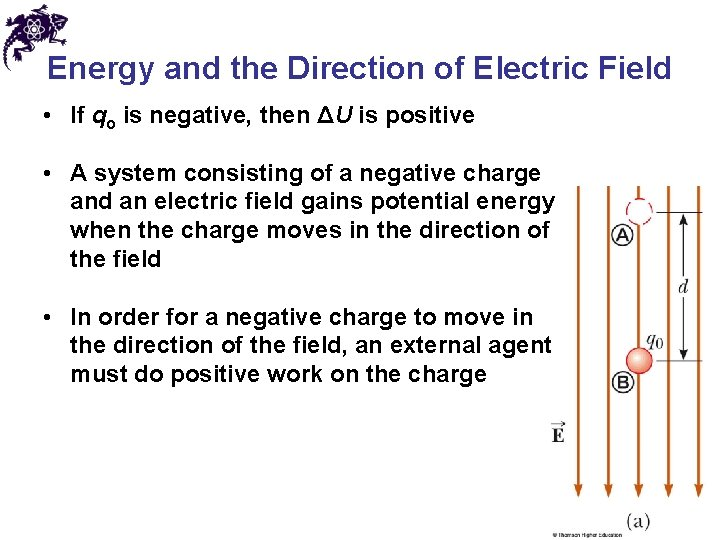Energy and the Direction of Electric Field • If qo is negative, then ΔU is positive • A system consisting of a negative charge and an electric field gains potential energy when the charge moves in the direction of the field • In order for a negative charge to move in the direction of the field, an external agent must do positive work on the chargeEnergy and Charge Movements • A positive (negative) charge gains (loses) electrical potential energy when it is moves in the direction opposite the electric field • If a charge is released in the electric field, it experiences a force and accelerates, gaining kinetic energy and losing an equal amount of electrical potential energy • When the electric field is directed downward, point B is at a lower potential than point A: a positive test charge moving from A to B loses electrical potential energyEquipotentials • Point B is at a lower potential than point A • Points B and C are at the same potential, since all points in a plane perpendicular to a uniform electric field are at the same electric potential • Equipotential surface is a continuous distribution of points having the same electric potentialChapter 22 Problem 23 An electric field is given by E = E 0 j^, where E 0 is a constant. Find the potential as a function of position, taking V = 0 at y = 0.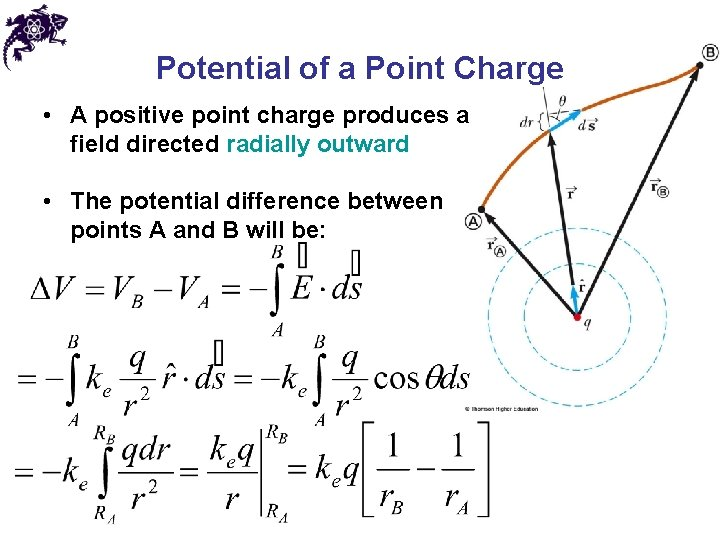Potential of a Point Charge • A positive point charge produces a field directed radially outward • The potential difference between points A and B will be: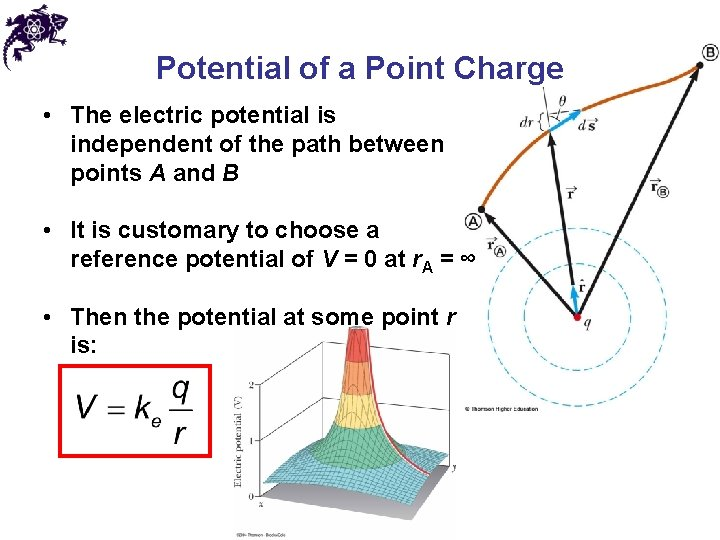Potential of a Point Charge • The electric potential is independent of the path between points A and B • It is customary to choose a reference potential of V = 0 at r. A = ∞ • Then the potential at some point r is: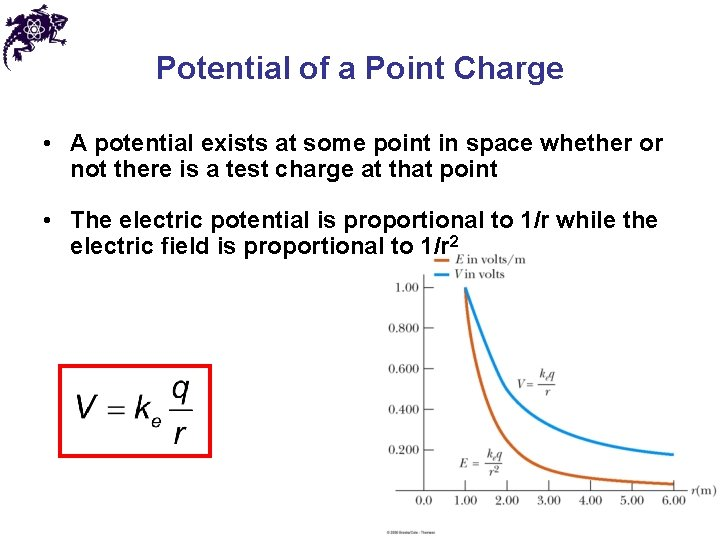Potential of a Point Charge • A potential exists at some point in space whether or not there is a test charge at that point • The electric potential is proportional to 1/r while the electric field is proportional to 1/r 2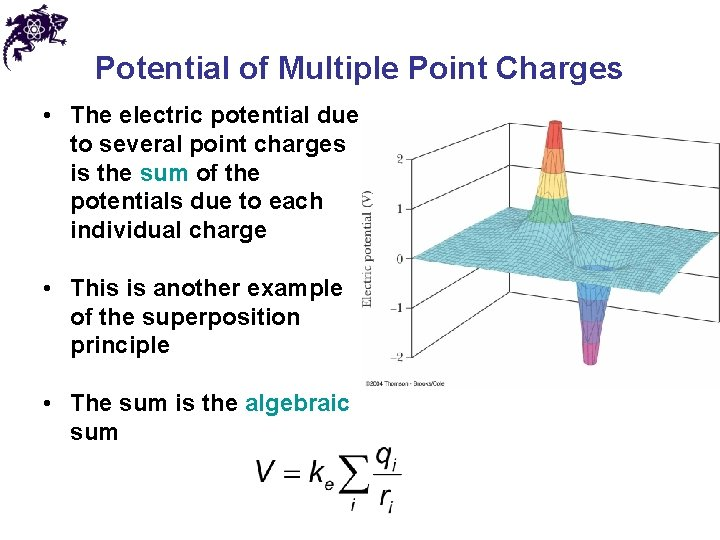Potential of Multiple Point Charges • The electric potential due to several point charges is the sum of the potentials due to each individual charge • This is another example of the superposition principle • The sum is the algebraic sum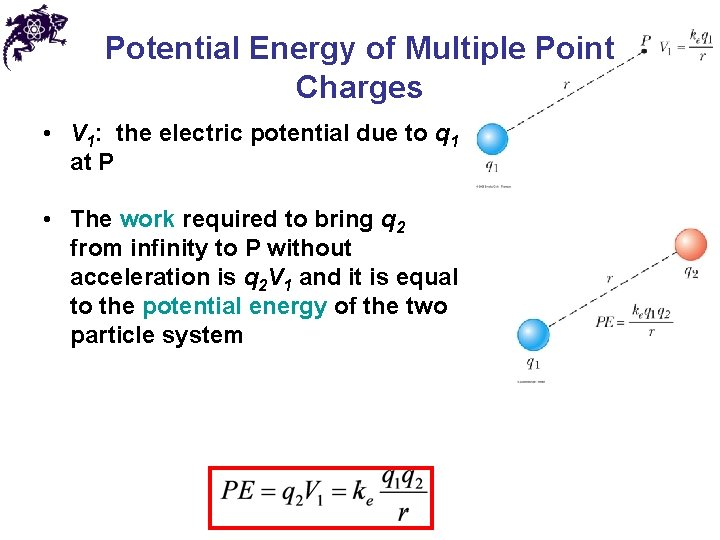Potential Energy of Multiple Point Charges • V 1: the electric potential due to q 1 at P • The work required to bring q 2 from infinity to P without acceleration is q 2 V 1 and it is equal to the potential energy of the two particle system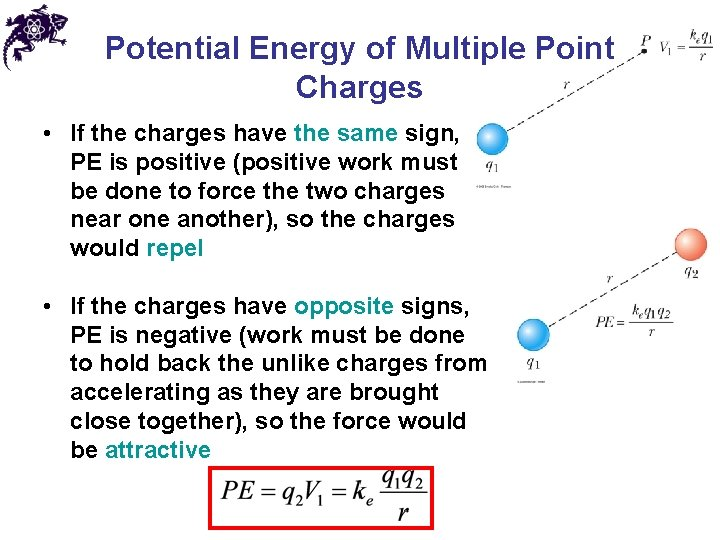Potential Energy of Multiple Point Charges • If the charges have the same sign, PE is positive (positive work must be done to force the two charges near one another), so the charges would repel • If the charges have opposite signs, PE is negative (work must be done to hold back the unlike charges from accelerating as they are brought close together), so the force would be attractiveFinding E From V • Assuming that the field has only an x component • Similar statements would apply to the y and z components • Equipotential surfaces must always be perpendicular to the electric field lines passing through them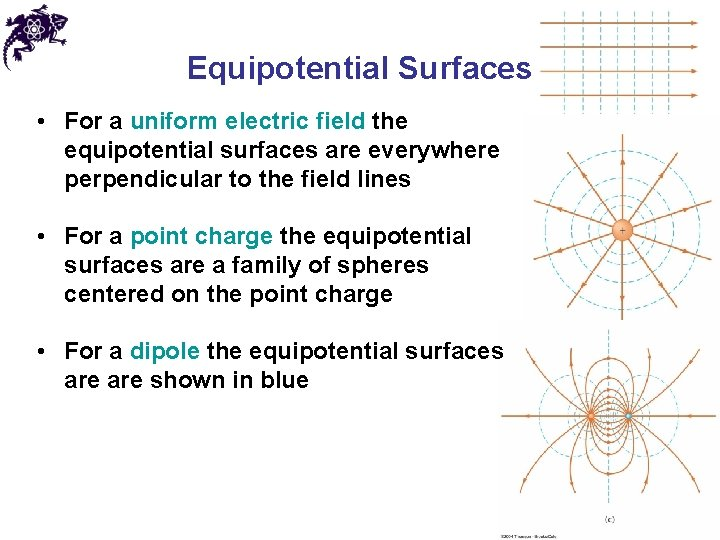Equipotential Surfaces • For a uniform electric field the equipotential surfaces are everywhere perpendicular to the field lines • For a point charge the equipotential surfaces are a family of spheres centered on the point charge • For a dipole the equipotential surfaces are shown in blue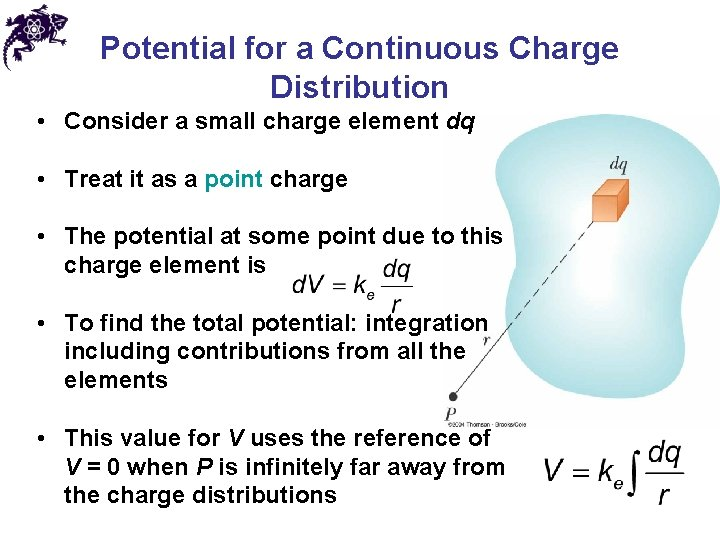Potential for a Continuous Charge Distribution • Consider a small charge element dq • Treat it as a point charge • The potential at some point due to this charge element is • To find the total potential: integration including contributions from all the elements • This value for V uses the reference of V = 0 when P is infinitely far away from the charge distributions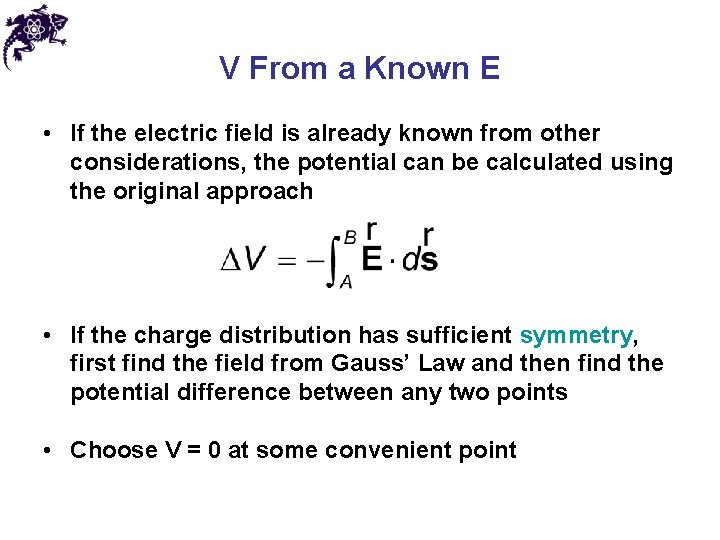V From a Known E • If the electric field is already known from other considerations, the potential can be calculated using the original approach • If the charge distribution has sufficient symmetry, first find the field from Gauss’ Law and then find the potential difference between any two points • Choose V = 0 at some convenient point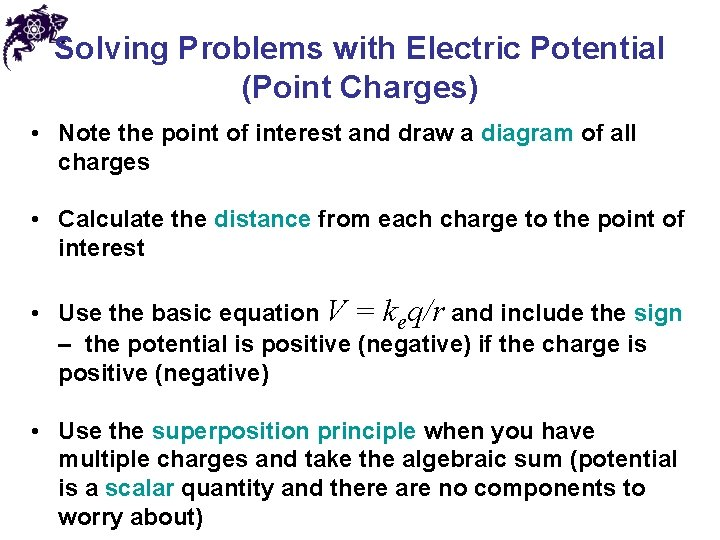Solving Problems with Electric Potential (Point Charges) • Note the point of interest and draw a diagram of all charges • Calculate the distance from each charge to the point of interest • Use the basic equation V = keq/r and include the sign – the potential is positive (negative) if the charge is positive (negative) • Use the superposition principle when you have multiple charges and take the algebraic sum (potential is a scalar quantity and there are no components to worry about)Solving Problems with Electric Potential (Continuous Distribution) • Define V = 0 at a point infinitely far away • If the charge distribution extends to infinity, then choose some other arbitrary point as a reference point • Each element of the charge distribution is treated as a point charge • Use integrals for evaluating the total potential at some point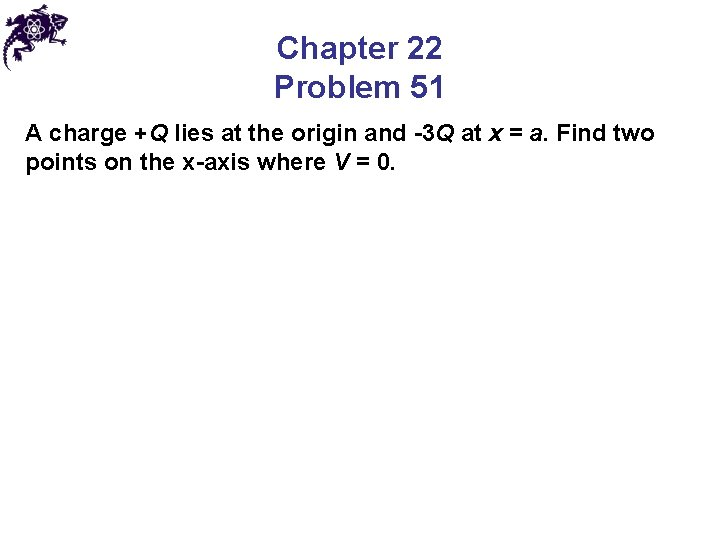Chapter 22 Problem 51 A charge +Q lies at the origin and -3 Q at x = a. Find two points on the x-axis where V = 0.Uniformly Charged Ring • P is located on the perpendicular central axis of the uniformly charged ring • The ring has a radius a and a total charge Q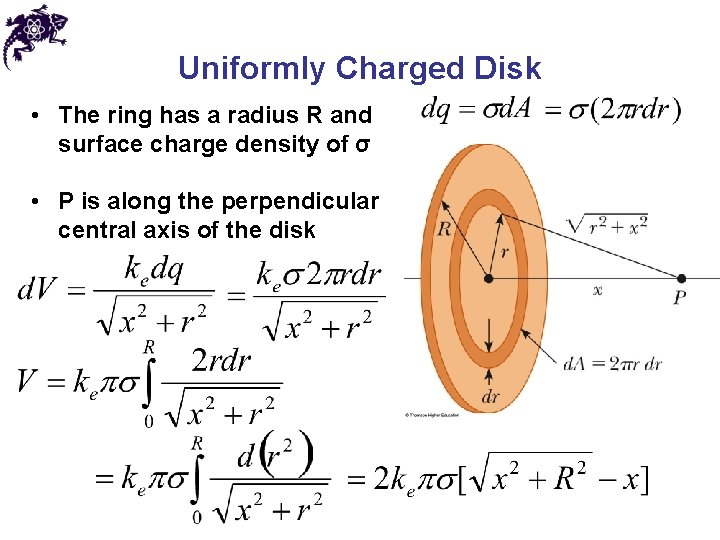Uniformly Charged Disk • The ring has a radius R and surface charge density of σ • P is along the perpendicular central axis of the disk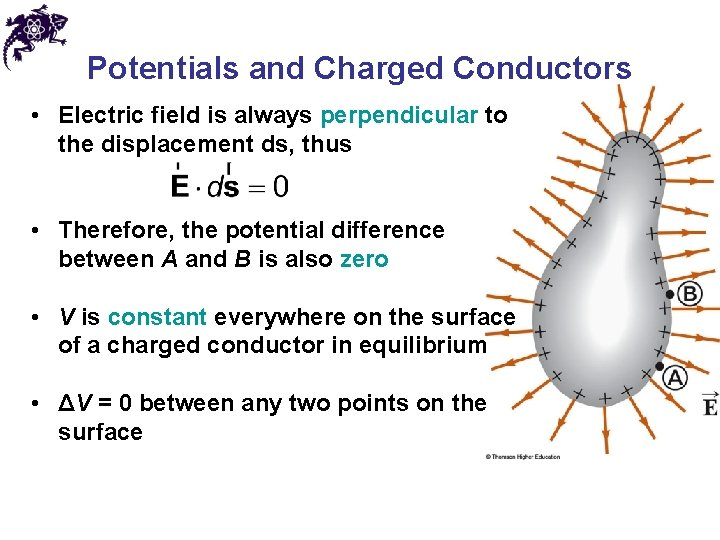Potentials and Charged Conductors • Electric field is always perpendicular to the displacement ds, thus • Therefore, the potential difference between A and B is also zero • V is constant everywhere on the surface of a charged conductor in equilibrium • ΔV = 0 between any two points on the surfacePotentials and Charged Conductors • The surface of any charged conductor in electrostatic equilibrium is an equipotential surface • The charge density is high (low) where the radius of curvature is small (large) • The electric field is large near the convex points (small radii of curvature) • Because E = 0 inside the conductor, the electric potential is constant everywhere inside the conductor and equal to the value at the surface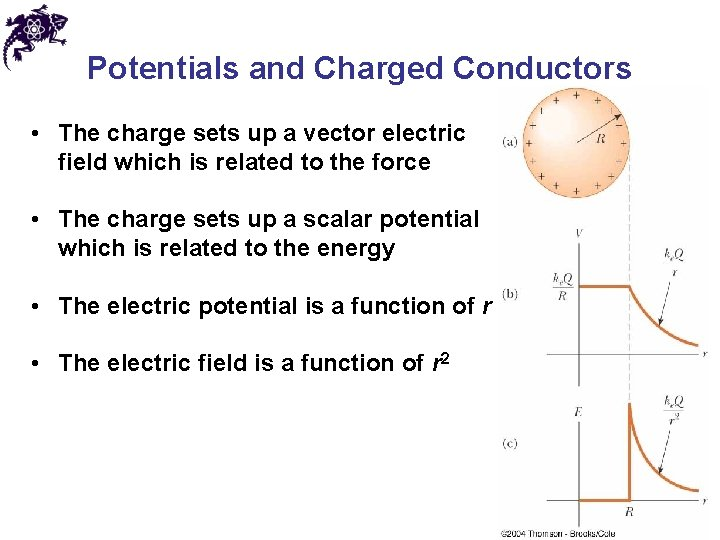Potentials and Charged Conductors • The charge sets up a vector electric field which is related to the force • The charge sets up a scalar potential which is related to the energy • The electric potential is a function of r • The electric field is a function of r 2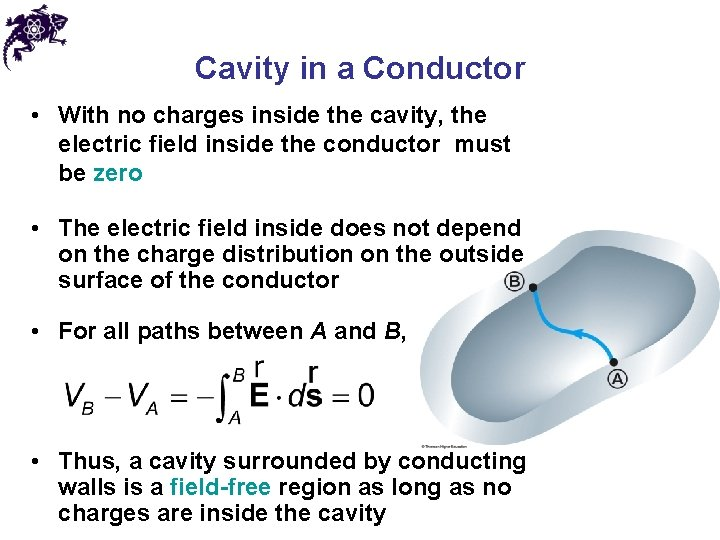Cavity in a Conductor • With no charges inside the cavity, the electric field inside the conductor must be zero • The electric field inside does not depend on the charge distribution on the outside surface of the conductor • For all paths between A and B, • Thus, a cavity surrounded by conducting walls is a field-free region as long as no charges are inside the cavityChapter 22 Problem 43 A sphere of radius R carries negative charge of magnitude Q, distributed in a spherically symmetric way. Find the escape speed for a proton at the sphere’s surface - that is, the speed that would enable the proton to escape to arbitrarily large distances starting at the sphere’s surface.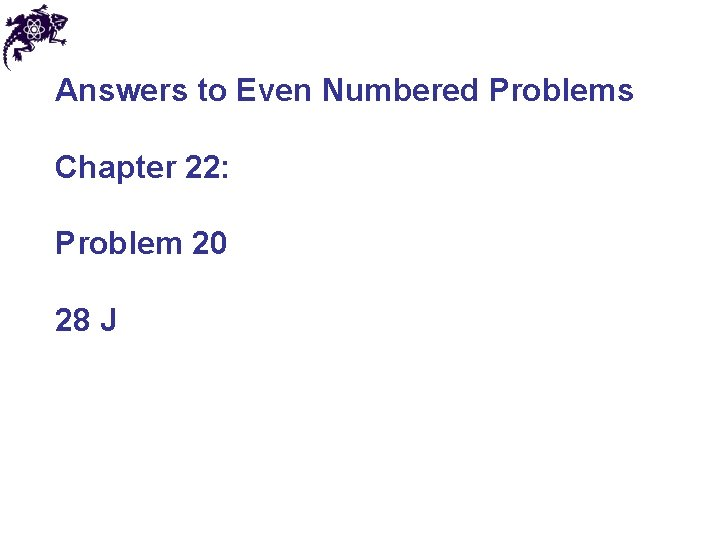Answers to Even Numbered Problems Chapter 22: Problem 20 28 J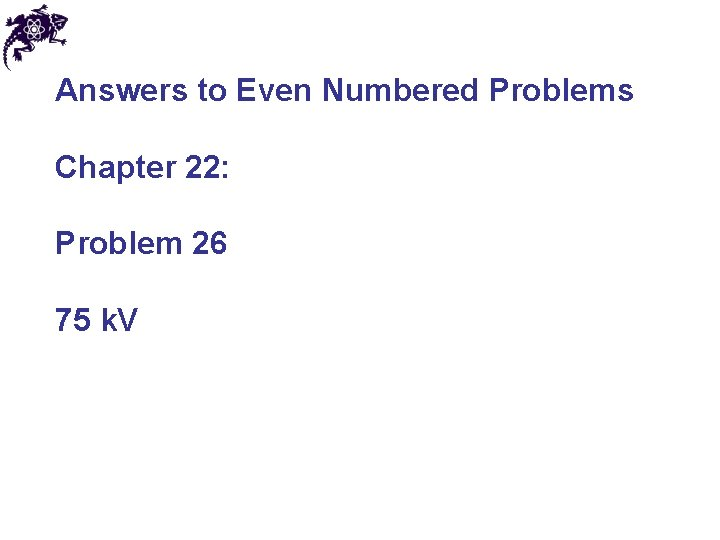Answers to Even Numbered Problems Chapter 22: Problem 26 75 k. V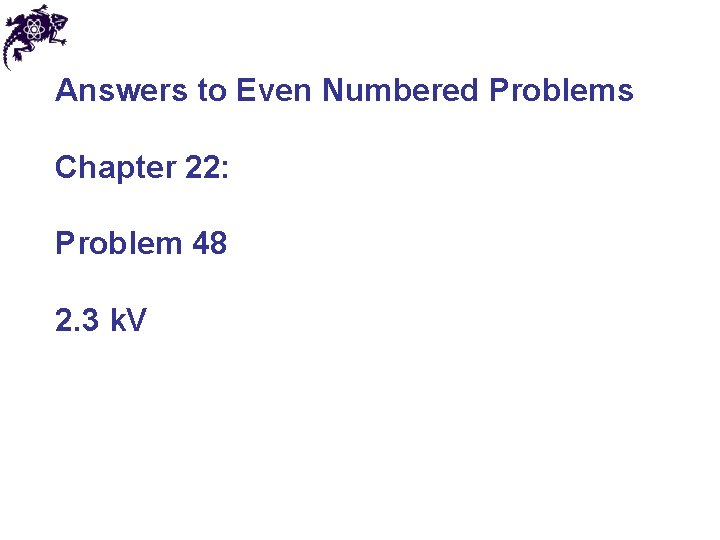Answers to Even Numbered Problems Chapter 22: Problem 48 2. 3 k. VAnswers to Even Numbered Problems Chapter 22: Problem 66 (a) 0, 1, and – 3 m (c) 0. 535 m and – 1. 87 m